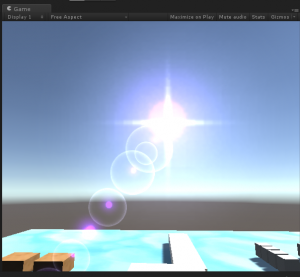# 浅谈 Unity3D 的 RenderQueue```Shader "Unlit/Overlayer2"
{
Properties
{
_MainTex ("Texture", 2D) = "white" {}
_Color("Color", Color) = (1,1,1,1)
}
{
Tags { "RenderType"="Opaque" "Queue"="Overlay+99999"}
//Blend SrcAlpha One
ZWrite Off
ZTest Off

Pass
{
CGPROGRAM
#pragma vertex vert
#pragma fragment frag
// make fog work
#pragma multi_compile_fog

#include "UnityCG.cginc"

struct appdata
{
float4 vertex : POSITION;
float2 uv : TEXCOORD0;
};

struct v2f
{
float2 uv : TEXCOORD0;
UNITY_FOG_COORDS(1)
float4 vertex : SV_POSITION;
};

sampler2D _MainTex;
float4 _MainTex_ST;
float4 _Color;

v2f vert (appdata v)
{
v2f o;
o.vertex = mul(UNITY_MATRIX_MVP, v.vertex);
o.uv = TRANSFORM_TEX(v.uv, _MainTex);
UNITY_TRANSFER_FOG(o,o.vertex);
return o;
}

fixed4 frag (v2f i) : SV_Target
{
// sample the texture
fixed a = tex2D(_MainTex, i.uv).r;
fixed4 col = fixed4(_Color.rgb * a, a);
// apply fog
UNITY_APPLY_FOG(i.fogCoord, col);
return col;
}
ENDCG
}
}
}

```

```using UnityEngine;
using System.Collections;

public class GetQueue : MonoBehaviour {
public int Queue;
// Use this for initialization
void Start () {

}

// Update is called once per frame
void Update () {
Renderer renderer = this.gameObject.GetComponent<Renderer> ();
Queue = renderer.sharedMaterial.renderQueue;
}
}

```